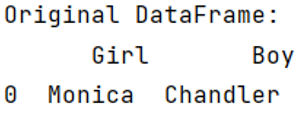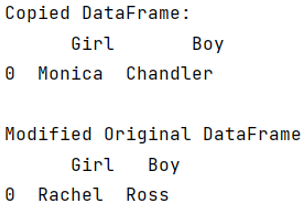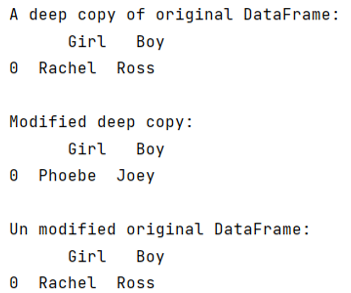# Why should we make a copy of a DataFrame in Pandas?

Learn, why should we make a copy of a DataFrame in Pandas? By Pranit Sharma Last updated : September 20, 2023

Pandas is a special tool that allows us to perform complex manipulations of data effectively and efficiently. Inside pandas, we mostly deal with a dataset in the form of DataFrame. DataFrames are 2-dimensional data structures in pandas. DataFrames consists of rows, columns, and the data.

## Problem statement

Learn, why should we make a copy of a DataFrame in Pandas? Also, write Python code to make a copy of a DataFrame in Pandas.

## Why should we make a copy of a DataFrame in Pandas?

During the analysis of data, we perform numerous operations on DataFrame, which can result in a drastic change in the original DataFrame. It is known that a copy is made by using the assignment operator in python but it is not true actually, using the assignment operator leads to sharing of references from one object to other. If we perform certain operations on these shared objects, it will modify the original object as it holds the same reference or it will raise an error. To overcome this problem, we should always make a copy of a DataFrame in pandas.

Let us understand with the help of an example.

## Create and print a dataframe

```# Importing pandas package
import pandas as pd

# Create DataFrame
df = pd.DataFrame({'Girl':['Monica'],'Boy':['Chandler']})
print("Original DataFrame:\n",df,"\n")
```

### Output

The output of the above program is:## Operations on dataframe without making a copy

Now let us perform some operations without making a copy of DataFrame and we will observe that it will modify the original DataFrame.

```# Assign df to df_copy
df_copy = df

# Display df_copy
print("Copied DataFrame:\n",df_copy,"\n")

# Update the value of group 1 and group 2
# in original DataFrame
df_copy['Girl']= 'Rachel'
df_copy['Boy'] = 'Ross'

print("Modified Original DataFrame\n",df)
```

### Output

The output of the above program is:## Create a deep copy of DataFrame and perform operations

Now we will make a deep copy of DataFrame and perform some operations and will observe that it will not modify the original DataFrame.

```# Using df.copy to make a copy
df_2 = df.copy(deep=True)

# Display df_2
print("A deep copy of original DataFrame:\n",df_2,"\n")

# Update values of deep copy
df_2['Girl'] = 'Phoebe'
df_2['Boy'] = 'Joey'

# Display updated deep copy and also original DataFrame
# which is not modified this time
print("Modified deep copy:\n",df_2,"\n")
print("Unmodified original DataFrame:\n",df)
```

### Output

The output of the above program is: